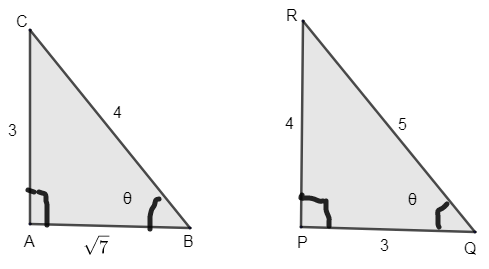Courses
Courses for Kids
Free study material
Offline Centres
MoreLast updated date: 06th Dec 2023
Total views: 382.5k
Views today: 4.82k

# If $12{{\cot }^{2}}\theta -31\cos ec+32=0$, then value of $\sin \theta$ is,(a) $\dfrac{3}{5}$or 1(b) $\dfrac{2}{3}$or $\dfrac{-2}{3}$(c) $\dfrac{4}{5}$or $\dfrac{3}{4}$(d) $\pm \dfrac{1}{2}$Verified
382.5k+ views
Hint: Substitute the trigonometric formula for ${{\cot }^{2}}\theta$. The entire equation becomes in terms of $\cos ec\theta$. Solve the equation formed and find the roots. $\sin \theta$ is the inverse of $\cos ec\theta$. Find the root and inverse it to get the value of $\sin \theta$.

Given the expression, $12{{\cot }^{2}}\theta -31\cos ec+32=0-(1)$
We know the trigonometric expression,
\begin{align} & \cos e{{c}^{2}}\theta -{{\cot }^{2}}\theta =1 \\ & \Rightarrow {{\cot }^{2}}\theta =\cos e{{c}^{2}}\theta -1 \\ \end{align}
Substitute the value of ${{\cot }^{2}}\theta$in equation (1),
\begin{align} & 12{{\cot }^{2}}\theta -31\cos e{{c}^{2}}\theta +32=0 \\ & 12\left( \cos e{{c}^{2}}\theta -1 \right)-31\cos ec\theta +32=0 \\ \end{align}
Opening the bracket and simplifying it,
\begin{align} & 12\cos e{{c}^{2}}\theta -12-31\cos ec\theta +32=0 \\ & 12\cos e{{c}^{2}}\theta -31\cos ec\theta +20=0-(2) \\ \end{align}
Now, equation (2) is in the form of a quadratic equation. We know a general quadratic equation is of the form $a{{x}^{2}}+bx+c=0$. Comparing both the general equation (1) and equation (2), we get,
$a=12,b=-31,c=20$
Now substitute these values in the quadratic form $\dfrac{-b\pm \sqrt{{{b}^{2}}-4ac}}{2a}$to get the roots.
\begin{align} & =\dfrac{-\left( -31 \right)\pm \sqrt{{{\left( -31 \right)}^{2}}-4\times 12\times 20}}{2\times 12} \\ & =\dfrac{31\pm \sqrt{961-960}}{24}=\dfrac{31\pm \sqrt{1}}{24} \\ & =\dfrac{31\pm 1}{24} \\ \end{align}
$\therefore$We get the roots as $\left( \dfrac{31+1}{24} \right)$and $\left( \dfrac{31-1}{24} \right)$$=\dfrac{32}{24}$and $\dfrac{30}{24}$.
$\therefore$The roots are $\dfrac{4}{3}$and $\dfrac{5}{4}$.
$\therefore$$\cos ec\theta =\dfrac{4}{3}$and $\cos ec\theta =\dfrac{5}{4}$.
We know, $\sin \theta =\dfrac{1}{\cos ec\theta }$
$\therefore \sin \theta =\dfrac{1}{\dfrac{4}{3}}$or $\dfrac{1}{\dfrac{5}{4}}$.
$\sin \theta =\dfrac{3}{4}$or $\dfrac{4}{5}$.
Hence, option (c) is correct.

Note: We got the value of $\sin \theta =\dfrac{3}{4}$or $\dfrac{4}{5}$.
Hence, we can find the value of $\cos \theta$ and $\tan \theta$.
$\sin \theta$ = opposite side/ Hypotenuse.
By Pythagoras theorem,$A{{B}^{2}}+A{{C}^{2}}=B{{C}^{2}}\Rightarrow AB=\sqrt{B{{C}^{2}}-A{{C}^{2}}}=\sqrt{{{4}^{2}}-{{3}^{2}}}$
$AB=\sqrt{16-9}=\sqrt{7}$
$P{{Q}^{2}}+P{{R}^{2}}\Rightarrow PQ=\sqrt{Q{{R}^{2}}-R{{P}^{2}}}=\sqrt{{{5}^{2}}-{{4}^{2}}}$
$PQ=\sqrt{25-16}=3$
$\tan \theta$= opposite side/ adjacent side $=\dfrac{3}{\sqrt{7}}$or $\dfrac{4}{3}$.
$\cos \theta$= adjacent side/ hypotenuse$=\dfrac{\sqrt{7}}{4}$or $\dfrac{3}{5}$.